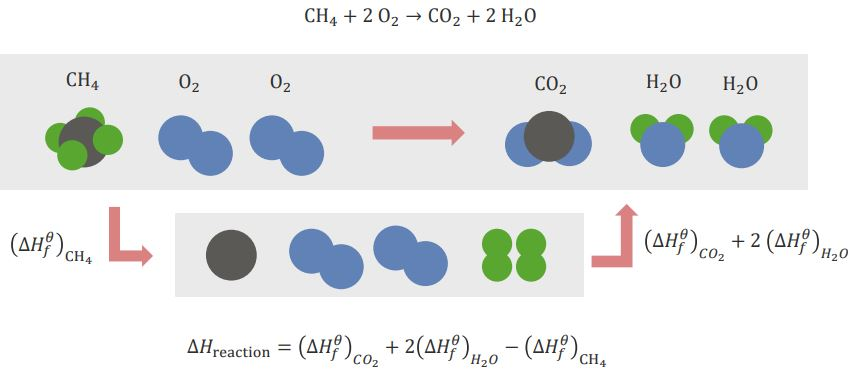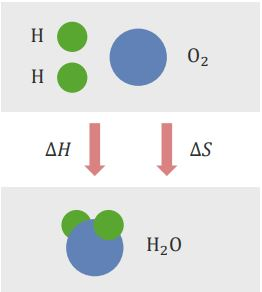Are you more of a visual learner? Check out our online video lectures and start your physics course now for free!Image: Heat. By: lisa-skorpion. License: CC BY-ND 2.0

Hess’s Law of Constant Heat Summation

Definition

Hess’s law of constant heat summation, or Hess’s law, states that no matter how many steps or stages are present in a reaction, the total enthalpy change for the entire reaction is the sum of each individual change. Enthalpy is a thermodynamic measurement assessing the total heat content of a system (see image below). It is equivalent to the internal energy of the system plus the product of the pressure and volume:

H = U + pV

Where:

H = enthalpy

U = internal energy

p = pressure

V = volume

In the reaction A → B → C, substance A is undergoing a reaction to become B, which then undergoes another reaction to become C. The change in enthalpy of the energy change is denoted by ΔH.

The ΔH for the total reaction is equal to the sum of the ΔH for the first reaction, plus the ΔH for the second reaction:

ΔHA͢͢͢͢͢ to B + ΔHB to C = ΔHA to C

Calculations Using Hess’s Law

Example of the calculation that takes place in a reaction:

CH4 + 2O2 → CO2 + 2H2O

Here, methane and oxygen react to form carbon dioxide and water. The elements need to be rearranged before the reaction can occur. This is done by breaking existing bonds and forming new bonds between elements.

CH4 (methane) needs to be broken down into its simple elements: C (carbon) and H (hydrogen). When this happens, an energy change occurs; this is referred to as (ΔHf)CH4. This is the standard energy of formation referring to standard elements present in nature. O2 (oxygen) does not need to be broken down into individual O elements since, in nature, a single O isn’t present. The standard form is O2.

To form the products, C and O have to combine to form CO2 (carbon dioxide) and H and O have to combine to form H2O (water). The enthalpies for forming these two products are (ΔHf)CO2 and (ΔHf)H2O, respectively.

The total enthalpy of the entire reaction is as follows:

ΔHreaction = (ΔHf)CO2 + 2(ΔHf)H2O – (ΔHf)CH4

The minus sign for the enthalpy for methane is due to the break-up of the bonds that are taking place:Image: Hess’s law. By: Lecturio.

Hess’s law is used to:

1. Determine the heat of formation
2. Determine the heat of transition
3. Determine the heat of hydration
4. Calculate bond energies

Gibbs Free Energy

DefinitionImage: Gibbs free energy. By: Lecturio.

Gibbs free energy is the energy that is associated with a chemical reaction that can be used to perform work. It conveys the amount of free energy that exists for a reaction to go forward (see image below). In general, a reaction wants to minimize energy use (enthalpy) and maximize entropy.

Entropy is the degree of disorder or randomness that exists in a system. The calculation of Gibbs free energy (ΔG) determines whether the reaction can occur spontaneously or not.

Enthalpy change (ΔH) and entropy change (ΔS) are competing reactions. Enthalpy seeks to be minimal, whereas entropy seeks to be maximal.

Entropy is also associated with temperature, since the disorder that exists in a system is dependent on temperature. The higher the temperature, the more disorder is present.

The relationship between Gibbs free energy (ΔG), enthalpy change (ΔH), and entropy change (ΔS) is as follows:

ΔG = ΔH – T ΔS

Where :

ΔG = Gibbs free energy

H = enthalpy

TS = random energy

ΔGsystem = –T ΔS total

Note the negative sign above; it denotes that enthalpy and entropy are opposing reaction concepts; as well, temperature (T) is measured using the Kelvin scale (K).

To Be Spontaneous or Not To Be Spontaneous; That is the Question

The reaction that needs to take place can occur either spontaneously (favorably) or nonspontaneously (unfavorably). This is determined by the calculation of Gibbs free energy (ΔG).

If ΔG < 0, then the reaction is considered to be a spontaneous reaction. If ΔG > 0, then the reaction is considered to be a non-spontaneous reaction.

Even if the reaction is exothermic (i.e., it requires no energy input), it may not occur if it lowers the entropy too much. As well, even if something is spontaneous, it does not necessarily occur quickly. Both of these concepts are not directly related, since they depend on different properties of the reaction.

Coefficient of Thermal Expansion

Definition

The coefficient of thermal expansion describes how the size of different types of matter can be affected (changed) by a change in temperature. Specifically, it measures the fractional change in size per degree change in temperature at a constant pressure. This change can be associated with linear (one-dimensional) change or volumetric (three-dimensional) change. When an object is heated (i.e., its temperature increases), the heated object is larger. Increasing heat will continue causing some expansion to occur based on the degree of Kelvin change in temperature.

Linear Thermal Expansion

An object has a linear dimension which, when heated, undergoes a linear expansion. The linear thermal expansion can only be measured in the solid state and is common in engineering applications. This change in thermal expansion in the linear (one-dimensional) direction is denoted as follows:

Lfinal = Linitial (1 + α ΔT)

Where:

Lfinal = final length

Linitial = initial length

α = coefficient of linear expansion

ΔT = temperature change

The coefficient of linear expansion is α = 1 / temperature; thus, the units for α are 1 / Kelvin.

Volumetric Thermal Expansion

An object has a volumetric dimension that, when heated, undergoes volumetric expansion. The volumetric thermal expansion can be measured for all condensed matter (liquid and solid states). This change in thermal expansion in the volumetric (three-dimensional) direction is denoted as follows:

Vfinal = Vinitial (1 + αv ΔT)

Where:

Vfinal = final volume

Vinitial = initial volume

αv = coefficient of volumetric expansion

ΔT = temperature change

The coefficient of volumetric expansion is αv = 3 α. This is because the volumetric coefficient is associated with three dimensions; thus, it is equal to three of the coefficient of linear expansions.

Simplifying Thermal Expansion Calculations

The thermal expansion equations for linear expansion and volumetric expansion can be stated in a different way based on a change in length and volume, respectively.

Linear Expansion

Lfinal = Linitial (1 + α ΔT)

Lfinal = Linitial + (Linitial α ΔT)

Lfinal = Linitial + ΔL

Thus, the change in length is ΔL = Linitial α ΔT.

Volumetric Expansion

Vfinal = Vinitial (1 + αv ΔT)

Vfinal = Vinitial + (Linitial αv ΔT)

Vfinal = Vinitial + ΔL

Thus, the change in volume is ΔV = Vinitial αv ΔT.

Applications of thermal expansion include the following:

1. In mechanical applications to fit parts together
2. In applications using the thermal expansion property, such as in bi-metal and mercury thermometers
Learn. Apply. Retain.
Your path to achieve medical excellence.
Study for medical school and boards with Lecturio.